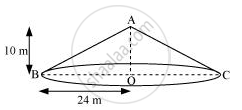# A conical tent is 10 m high and the radius of its base is 24 m. Find (i) slant height of the tent (ii) cost of the canvas required to make the tent, if the cost of 1 m2 canvas is Rs 70. - Mathematics

A conical tent is 10 m high and the radius of its base is 24 m. Find

(i) slant height of the tent

(ii) cost of the canvas required to make the tent, if the cost of 1 m2 canvas is Rs 70.

["Assume "pi=22/7]

#### Solution(i) Let ABC be a conical tent.

Height (h) of conical tent = 10 m

Radius (r) of conical tent = 24 m

Let the slant height of the tent be l.

In ΔABO,

AB2 = AO2 + BO2

l2 = h2 + r2

= (10 m)2 + (24 m)2

= 676 m2

∴ l = 26 m

Therefore, the slant height of the tent is 26 m.

(ii) CSA of tent = πrl

=(22/7xx24xx26)m^2

=13728/7m^2

Cost of 1 m2 canvas = Rs 70

"Cost of "13728/7m^2" canvas "=Rs.(13728/7xx70)

= Rs. 137280

Therefore, the cost of the canvas required to make such a tent is Rs 137280.

Concept: Surface Area of a Right Circular Cone
Is there an error in this question or solution?
Chapter 13: Surface Area and Volumes - Exercise 13.3 [Page 221]

#### APPEARS IN

NCERT Class 9 Maths
Chapter 13 Surface Area and Volumes
Exercise 13.3 | Q 4 | Page 221

Share